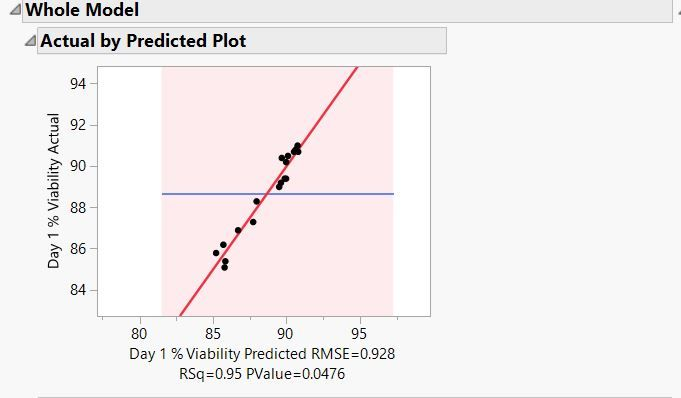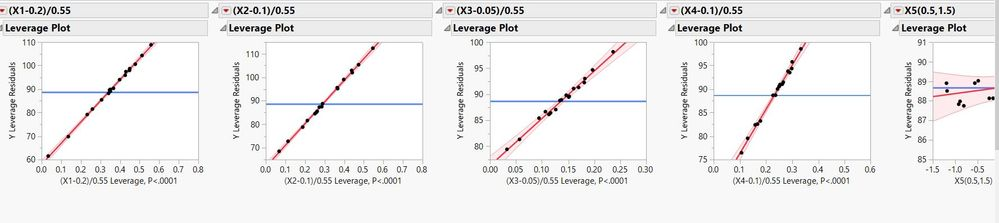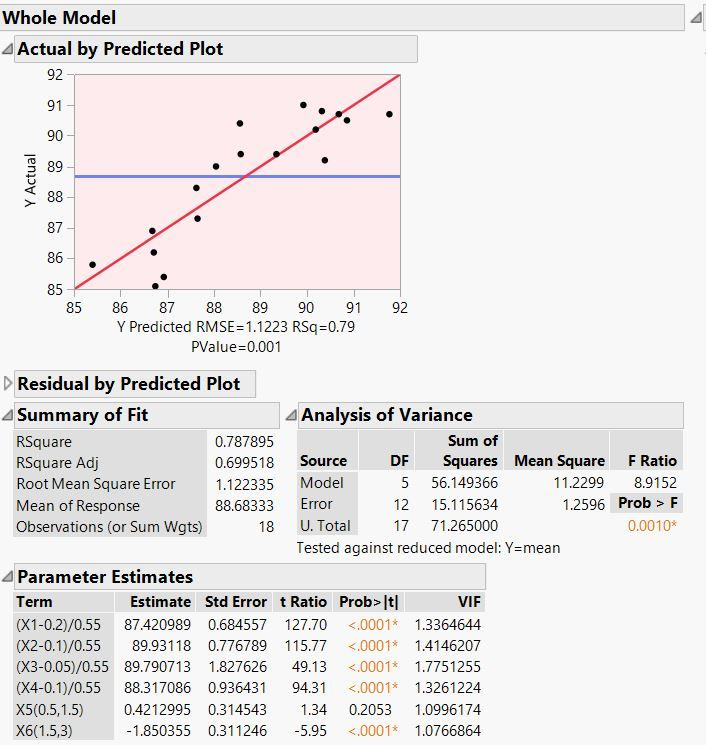The World Statistics Day celebration continues here in the Community. We all need reliable data for sound decision making. Do you have a data source that you trust most? Head over to Discussions to tell us about it.
Choose Language Hide Translation Bar
Highlighted

## ERROR: 0.05 significant Curve shaded error plot even though my model is significant with high R2 value

I fit model in JMP. Everything but my graph looks very strange.

THe model is significant at 0.0476

RSquare 0.951662
Root Mean Square Error 0.928013
Mean of Response 88.68333
Observations (or Sum Wgts) 18

The pink area seems to cover everything as an error. Has anyone else Encounter this?1 ACCEPTED SOLUTION

Accepted Solutions
Highlighted

## Re: ERROR: 0.05 significant Curve shaded error plot even though my model is significant with high R2 value

Ah, the mixture situation explains alot of this. You are fitting a Scheffe model. They will typically have more error associated with the prediction because of the built-in collinearity of the mixture factors.

Expand your y-axis scale on the observed versus predicted plot. I think you will see the error bounds appear. There will likely be high error because you really do not have many significant terms in this model. Perhaps only one term, X6.

The significance of your X1-X4 terms is misleading. The p-values are associated with the test comparing the parameter estimate to 0. But you are fitting a Scheffe model. The parameter estimate for a Scheffe mixture term is the combination of the mean of the data AND the effect of the component. So a more meaningful test would be to compare the parameter to the overall mean (88.68 for your scenario). Considering that, you will see that none of the X1 through X4 terms look to be "significant". You can verify by adding the 95% confidence interval on for each of the parameter estimates. If the confidence intervals for X1 - X4 contain the overall mean, that component is not having much of an effect.

Stated another way, it looks like you are getting about the same response everywhere within the mixture space. X5 does not seem to have an impact, but X6 does.

Dan Obermiller
8 REPLIES 8
Highlighted

## Re: ERROR: 0.05 significant Curve shaded error plot even though my model is significant with high R2 value

I think a few more details might be needed. Typically, these strange types of results occur because of very high multi-collinearity in the model terms.

What do the leverage plots look like?

If you right-click on the Parameter Estimates table and add the VIF column, what do those numbers look like?

Dan Obermiller
Highlighted

## Re: ERROR: 0.05 significant Curve shaded error plot even though my model is significant with high R2 value

Hi Dan, Thank you for your quick response.

The Leverage Plots all looks pretty good. Example Pics attached here.The VIF Is pretty high (>5):

VIF
12.19448
11.013741
16.435892
28.099305
1.1546501
1.2200138
7.8651502
14.560553
5.9118511
10.052586
8.2589787
5.4652854
5.4652854
1.0590402

However, even when I step thru the model to eliminate some of these factors, a refined version of the model with VIF less than 3 still has the same shaded plot. (attached here). This is also a mixture study so X1, 2, 3, and X4 are inherently correlated (add up to a sum of 100%).Highlighted

## Re: ERROR: 0.05 significant Curve shaded error plot even though my model is significant with high R2 value

Ah, the mixture situation explains alot of this. You are fitting a Scheffe model. They will typically have more error associated with the prediction because of the built-in collinearity of the mixture factors.

Expand your y-axis scale on the observed versus predicted plot. I think you will see the error bounds appear. There will likely be high error because you really do not have many significant terms in this model. Perhaps only one term, X6.

The significance of your X1-X4 terms is misleading. The p-values are associated with the test comparing the parameter estimate to 0. But you are fitting a Scheffe model. The parameter estimate for a Scheffe mixture term is the combination of the mean of the data AND the effect of the component. So a more meaningful test would be to compare the parameter to the overall mean (88.68 for your scenario). Considering that, you will see that none of the X1 through X4 terms look to be "significant". You can verify by adding the 95% confidence interval on for each of the parameter estimates. If the confidence intervals for X1 - X4 contain the overall mean, that component is not having much of an effect.

Stated another way, it looks like you are getting about the same response everywhere within the mixture space. X5 does not seem to have an impact, but X6 does.

Dan Obermiller
Highlighted

## Re: ERROR: 0.05 significant Curve shaded error plot even though my model is significant with high R2 value

Thank you Dan. That makes sense.

Given this interpretation, my model is still significant at alpha <0.05 correct? Even if it is just base on the value of X6?

Thanks again

Highlighted

## Re: ERROR: 0.05 significant Curve shaded error plot even though my model is significant with high R2 value

You are correct that the overall model is likely significant, based on the X6 term.

Realize that because of the issues with the main effects of a Scheffe model, the overall model test may show as significant even if the model is not.

Dan Obermiller
Highlighted

## Re: ERROR: 0.05 significant Curve shaded error plot even though my model is significant with high R2 value

Hi Dan,

I have a follow up to the Scheffe  model fit issue in the fit-model platform. Is there a way to fit this model appropriately where the parameter estimate is comparing the estimate to null=mean instead of comparing it to null=0?
In other word, is there a better way to fit this model to get a more correct output and transfer equation?

Thank you,

Highlighted

## Re: ERROR: 0.05 significant Curve shaded error plot even though my model is significant with high R2 value

A colleague of mine wrote a script to provide the proper testing. However, I always caution anyone working with formulations: what will you do with this information? Suppose a component is not significant. What does that really mean? Does it mean you could remove that component from the formulation? No, it does not mean that. You MIGHT be able to remove it from the formulation, but Scheffe models are NOT cause-and-effect. So, use this with caution. Finally, this script will add columns to an existing Parameter Estimates table (even if that report is closed). You will see testing on all of the model effects, the report is only valid for the main effects.

``````Names Default to Here( 1 );

// find current Fit Least Squares platform
window = Get Window List();
n windows = N Items( window );
For( w = 1, w <= n windows, w++,
window ref = Get Window( w );
title = window ref << Get Window Title;
If( Contains( title, "Fit Least Squares" ),
Break();
);
);

// obtain values for proper t-test of estimates
mean = window ref["Summary of Fit"][NumberColBox(1)] << Get( 4 );
err df = window ref["Analysis of Variance"][NumberColBox(1)] << Get( 2 );
estimate = window ref["Parameter Estimates"][NumberColBox(1)] << Get As Matrix;
std err = window ref["Parameter Estimates"][NumberColBox(2)] << Get As Matrix;

// compute t-ratios
t ratio = (estimate - mean) :/ std err;

// compute p-value for each t-ratio
p val = 2 * (1 - Students t Distribution( Abs( t ratio ), err df ));

// add proper test to report
window ref["Parameter Estimates"][TableBox(1)]
<< Append( Number Col Box( "Proper t Ratio", t ratio ) )
<< Append( Number Col Box( "Proper Prob>|t|", p val ) );

window ref["Parameter Estimates"][NumberColBox("Proper Prob>|t|")] << Set Format( 8, 97) << Set Conditional Format( "PValue" );

window ref["Parameter Estimates"] << Append(
Text Box( "Proper tests valid for linear terms only!" );
);
``````
Dan Obermiller
Highlighted